﻿ 广州圣丰索菲特大酒店团购_广州圣丰索菲特大酒店预订 【114票务网】

# 上海快3上海快3 > 酒店 > 广州> 广州圣丰索菲特大酒店

### 广州圣丰索菲特大酒店1032

4.8分 /（247人评价）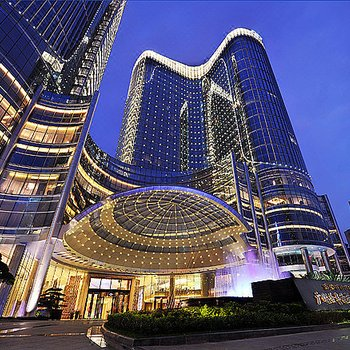••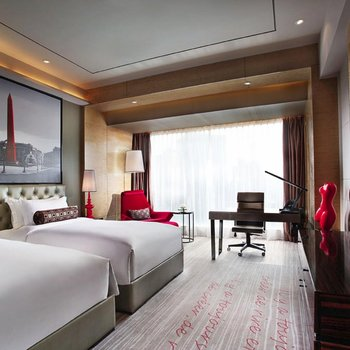•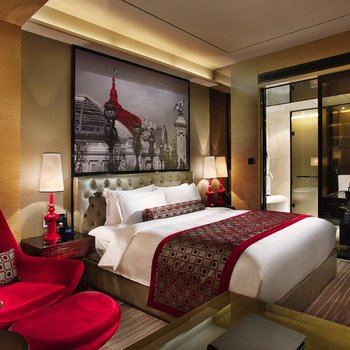•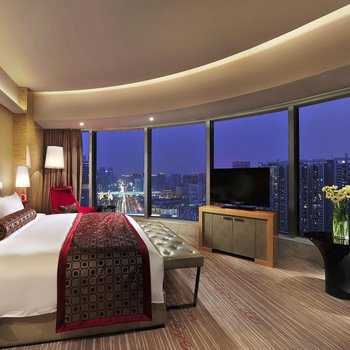•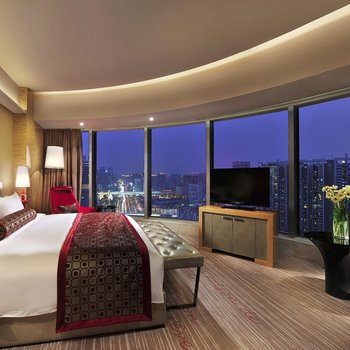•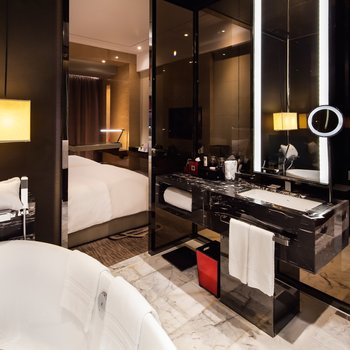••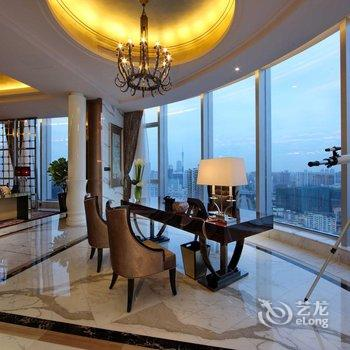••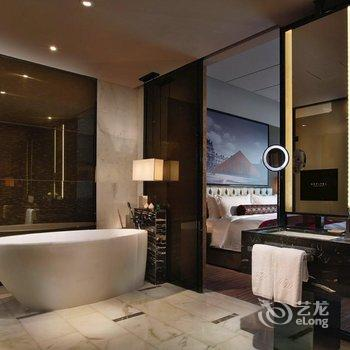••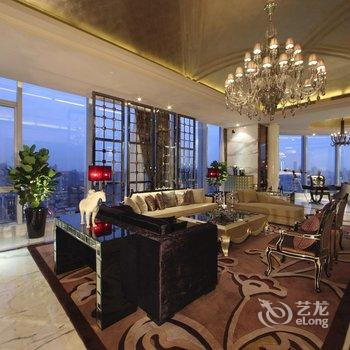•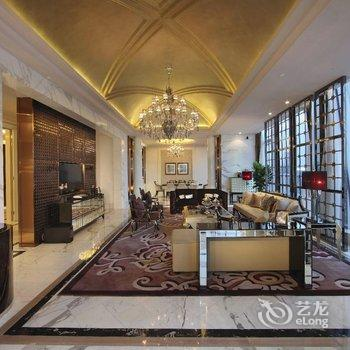•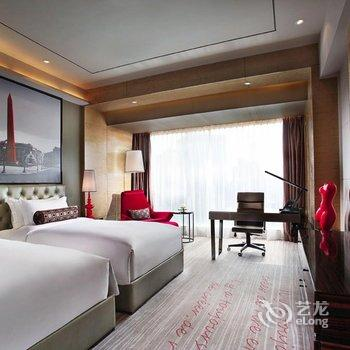•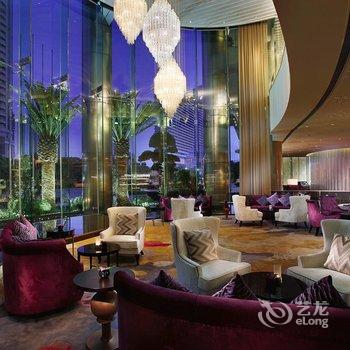•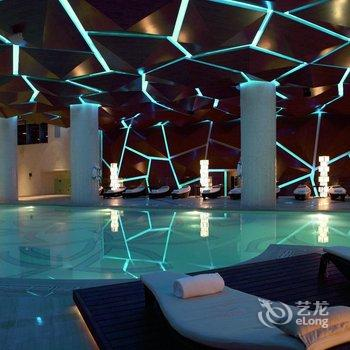•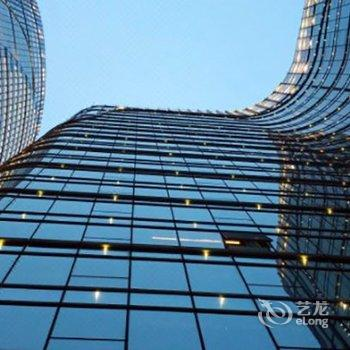•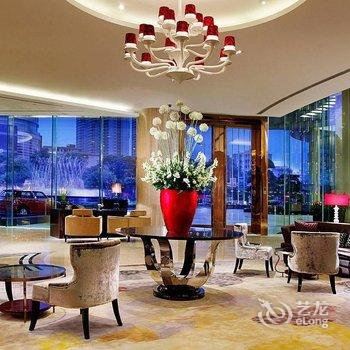•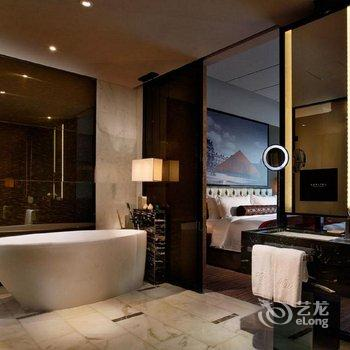•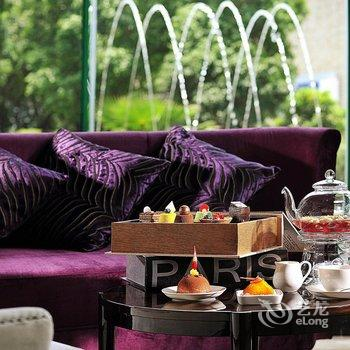•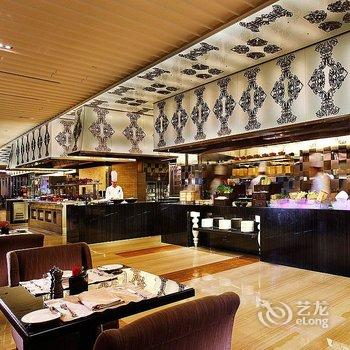•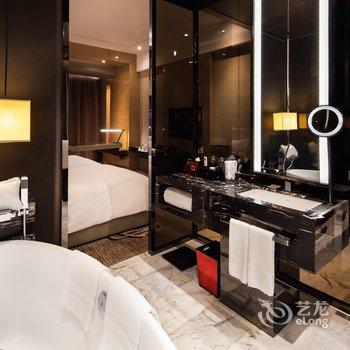•••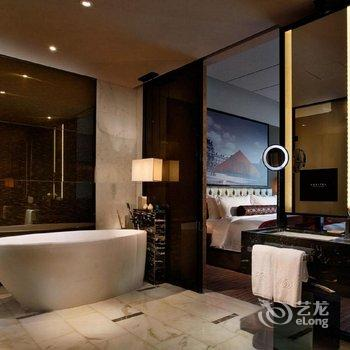••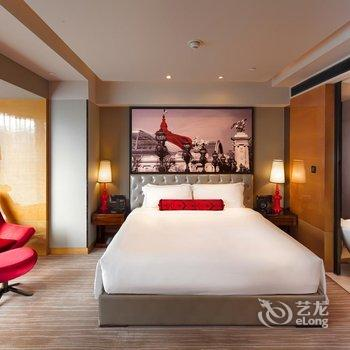•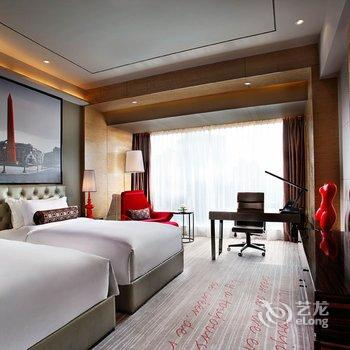•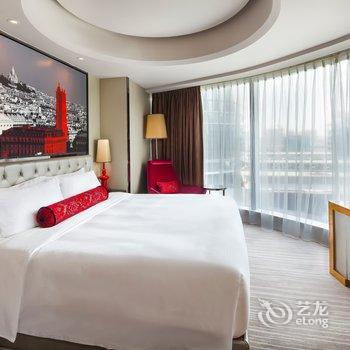••••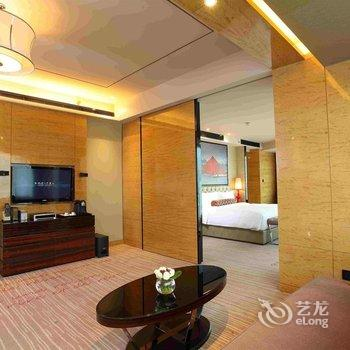•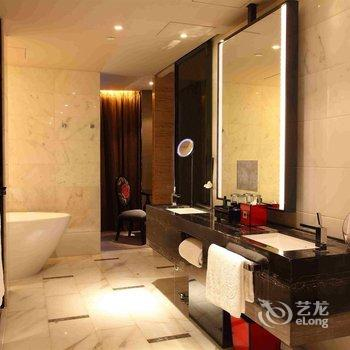•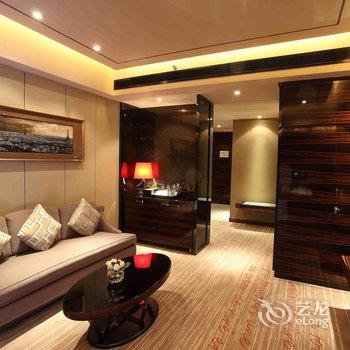••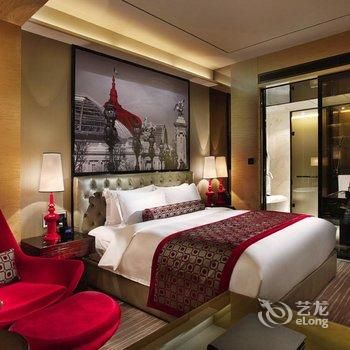•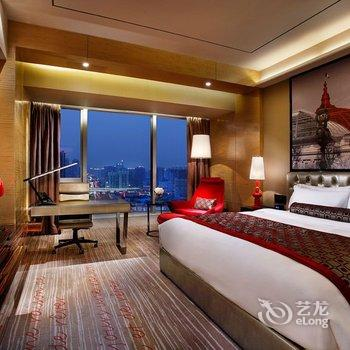•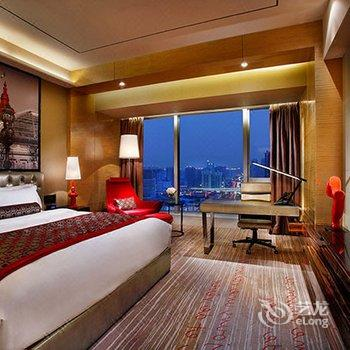•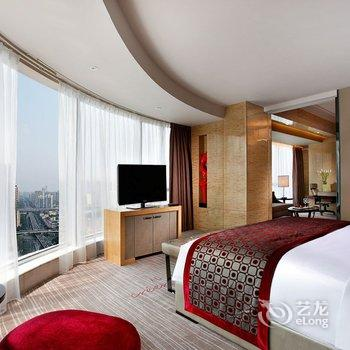•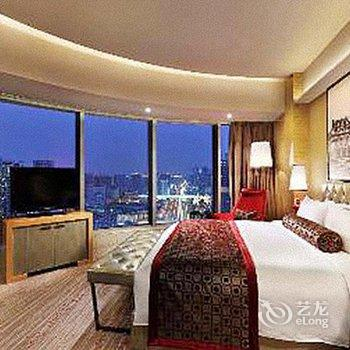•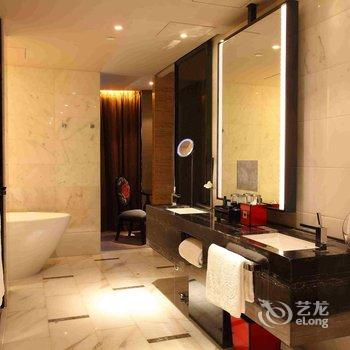•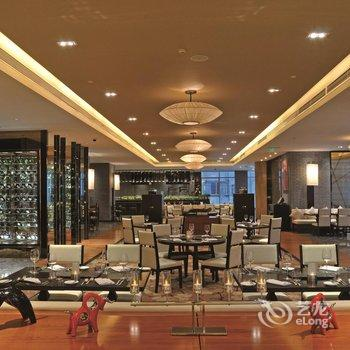••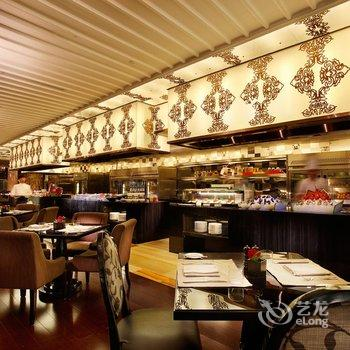••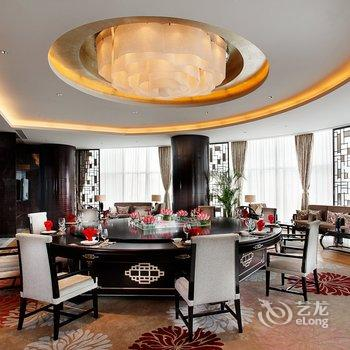•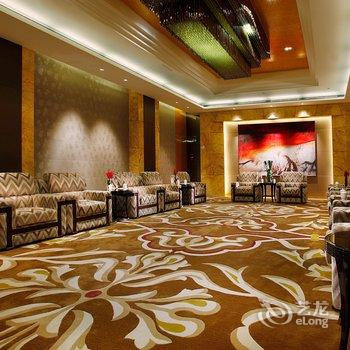•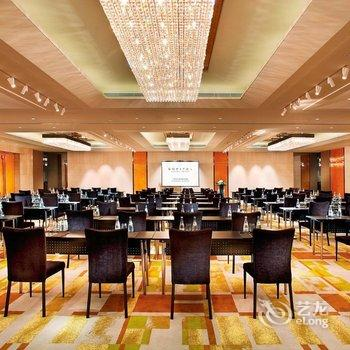•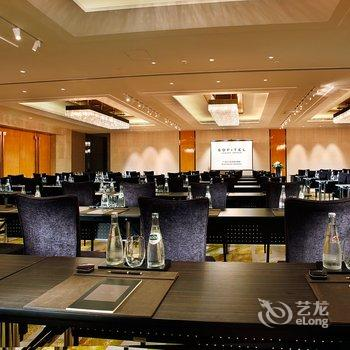•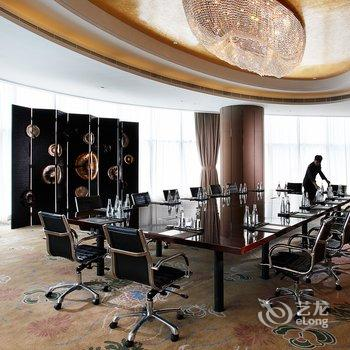•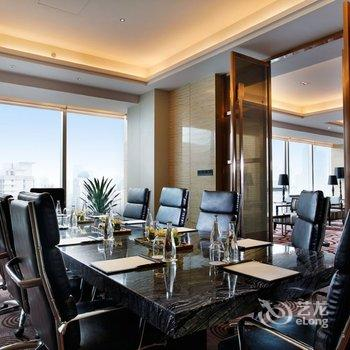••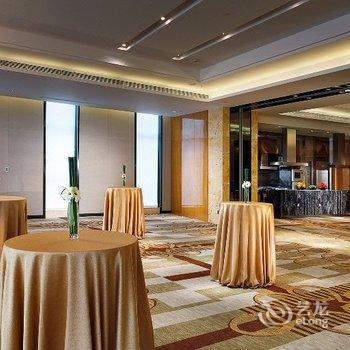••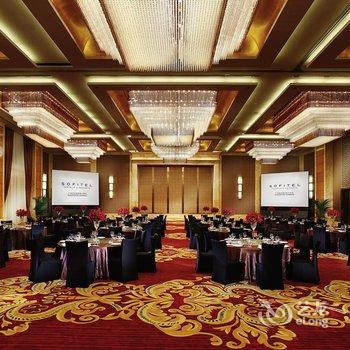•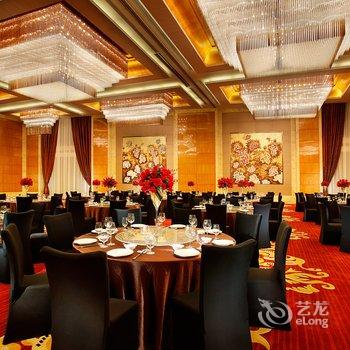•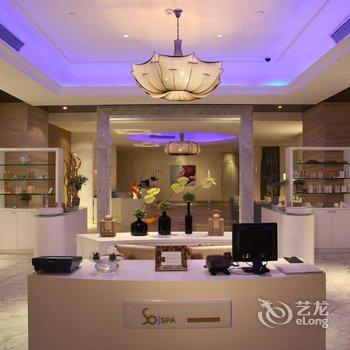•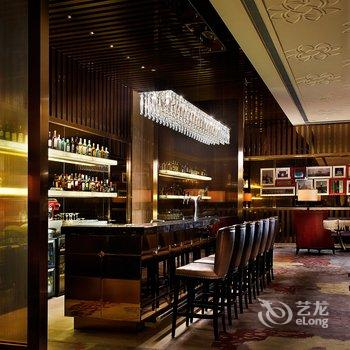•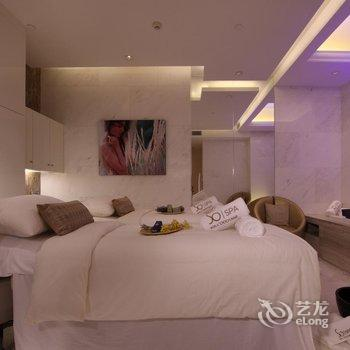•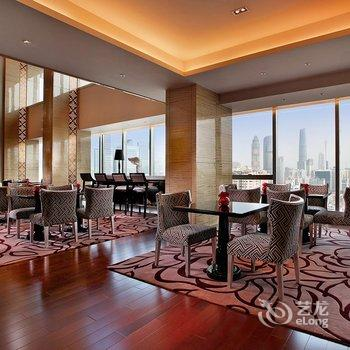•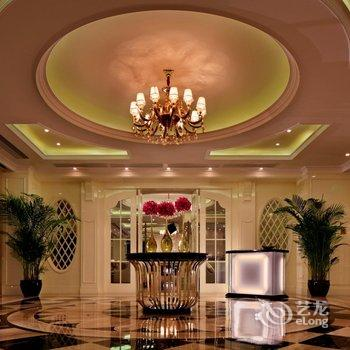•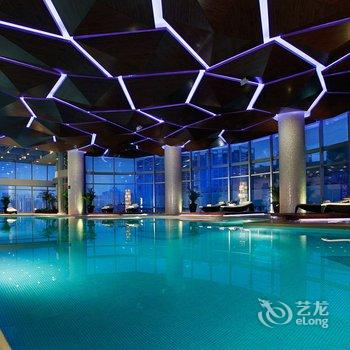••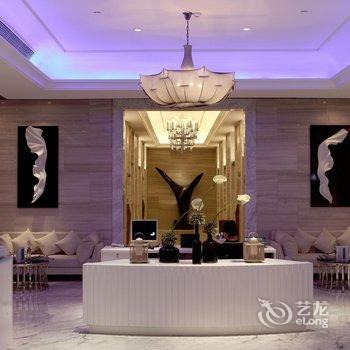•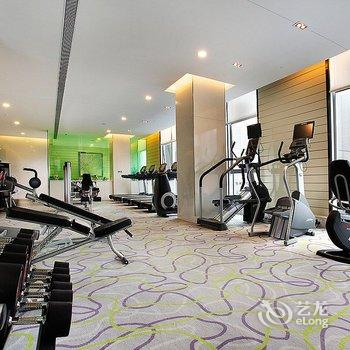•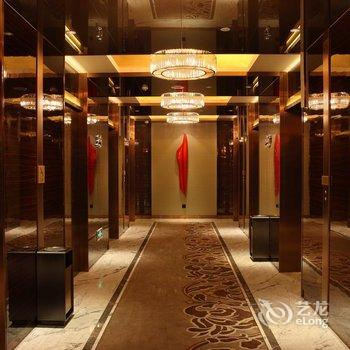•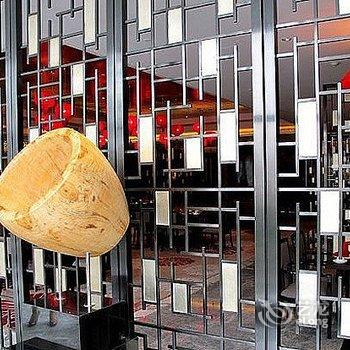•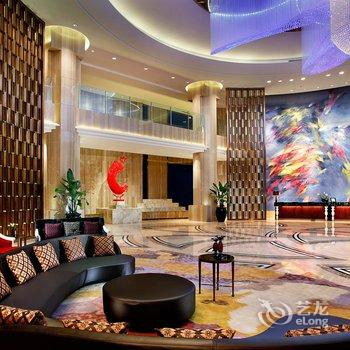•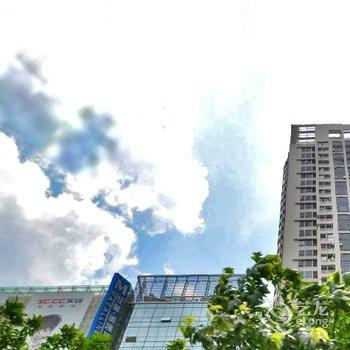•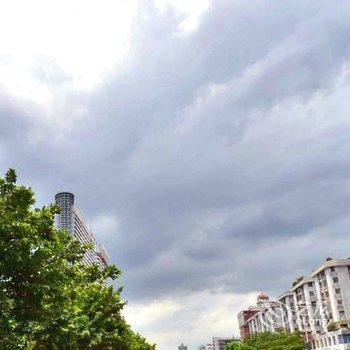•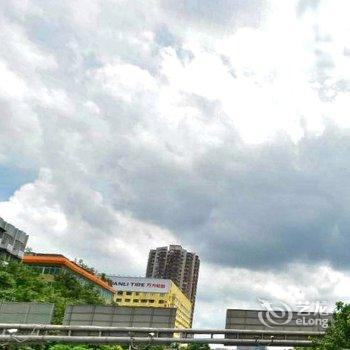•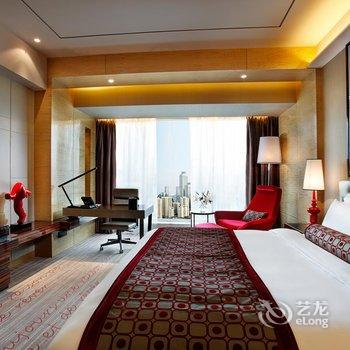•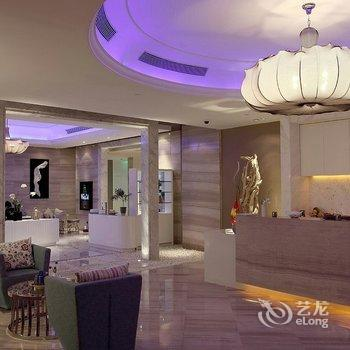•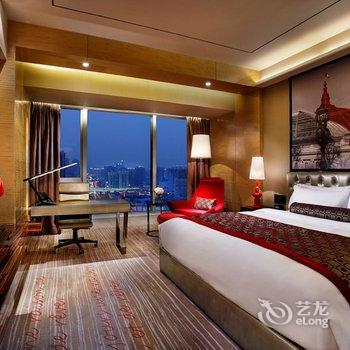•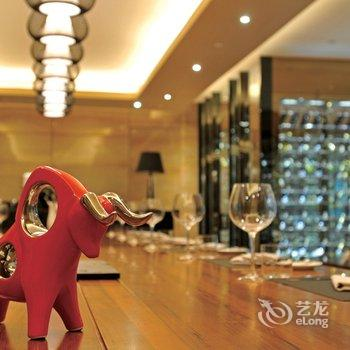•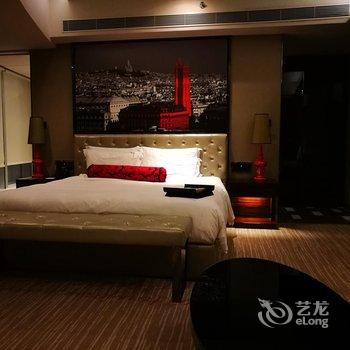#### 高级大床房

• 含双早（预付A）
• 大床2米
• 有窗
• 免费无线
• ￥1166.55
• 有房
• 预付
• 预订
• 含双早（微信专享）
• 大床2米
• 有窗
• 免费无线
• ￥1166.55
• 有房
• 预付
• 预订#### 高级双床房

• 含双早（预付A）
• 双床1.2米
• 有窗
• 免费无线
• ￥1166.55
• 有房
• 预付
• 预订
• 含双早（微信专享）
• 双床1.2米
• 有窗
• 免费无线
• ￥1166.55
• 有房
• 预付
• 预订#### 豪华大床房

• 含双早（预付A）
• 大床2米
• 有窗
• 免费无线
• ￥1388.75
• 有房
• 预付
• 预订
• 含双早（微信专享）
• 大床2米
• 有窗
• 免费无线
• ￥1388.75
• 有房
• 预付
• 预订
• 标准价
• 大床2米
• 有窗
• 免费无线
• ￥1488.1
• 有房
• 预付
• 预订
• 标准价
• 大床2米
• 有窗
• 免费无线
• ￥1523.81
• 有房
• 预付
• 预订
• 标准价
• 大床2米
• 有窗
• 免费无线
• ￥1540.48
• 有房
• 预付
• 预订
• 标准价
• 大床2米
• 有窗
• 免费无线
• ￥1623.81
• 有房
• 预付
• 预订
• 标准价
• 大床2米
• 有窗
• 免费无线
• ￥1660.71
• 有房
• 预付
• 预订
• 标准价
• 大床2米
• 有窗
• 免费无线
• ￥1791.67
• 有房
• 预付
• 预订#### 会所高级房

• (大床)(特惠房)
• 大/双床
• 未知
• 免费无线
• ￥1566.65
• 有房
• 预付
• 预订
• 标准价
• 大/双床
• 未知
• 免费无线
• ￥1574
• 有房
• 担保
• 预订
• 标准价
• 大/双床
• 未知
• 免费无线
• ￥1574
• 有房
• 担保
• 预订
• 标准价
• 大/双床
• 未知
• 免费无线
• ￥1574
• 有房
• 担保
• 预订
• 标准价
• 大/双床
• 未知
• 免费无线
• ￥1574
• 有房
• 担保
• 预订
• 标准价
• 大/双床
• 未知
• 免费无线
• ￥1654.43
• 有房
• 预付
• 预订
• 标准价
• 大/双床
• 未知
• 免费无线
• ￥1678.57
• 有房
• 预付
• 预订
• 标准价
• 大/双床
• 未知
• 免费无线
• ￥1684.52
• 有房
• 预付
• 预订
• 标准价
• 大/双床
• 未知
• 免费无线
• ￥1721.43
• 有房
• 预付
• 预订
• 标准价
• 大/双床
• 未知
• 免费无线
• ￥1721.43
• 有房
• 预付
• 预订
• 标准价
• 大/双床
• 未知
• 免费无线
• ￥1761.09
• 有房
• 预付
• 预订
• 标准价
• 大/双床
• 未知
• 免费无线
• ￥1773.32
• 有房
• 预付
• 预订
• 标准价
• 大/双床
• 未知
• 免费无线
• ￥1816.65
• 有房
• 预付
• 预订
• 标准价
• 大/双床
• 未知
• 免费无线
• ￥1883.31
• 有房
• 预付
• 预订
• 标准价
• 大/双床
• 未知
• 免费无线
• ￥1892.2
• 有房
• 预付
• 预订
• 标准价
• 大/双床
• 未知
• 免费无线
• ￥2006.65
• 有房
• 预付
• 预订
• 标准价
• 大/双床
• 未知
• 免费无线
• ￥2015.54
• 有房
• 预付
• 预订
• 标准价
• 大/双床
• 未知
• 免费无线
• ￥2021.09
• 有房
• 预付
• 预订
• 标准价
• 大/双床
• 未知
• 免费无线
• ￥2229.98
• 有房
• 预付
• 预订
• 标准价
• 大/双床
• 未知
• 免费无线
• ￥2241.09
• 有房
• 预付
• 预订
• 标准价
• 大/双床
• 未知
• 免费无线
• ￥2252.2
• 有房
• 预付
• 预订
• 标准价
• 大/双床
• 未知
• 免费无线
• ￥2566.75
• 有房
• 预付
• 预订
• (特惠促销)
• 大/双床
• 未知
• 免费无线
• ￥2733.31
• 有房
• 预付
• 预订
• 标准价
• 大/双床
• 未知
• 免费无线
• ￥2900.08
• 有房
• 预付
• 预订
• (特惠促销)
• 大/双床
• 未知
• 免费无线
• ￥2955.53
• 有房
• 预付
• 预订
• (特惠促销)
• 大/双床
• 未知
• 免费无线
• ￥3177.75
• 有房
• 预付
• 预订
• 标准价
• 大/双床
• 未知
• 免费无线
• ￥3233.41
• 有房
• 预付
• 预订
• 标准价
• 大/双床
• 未知
• 免费无线
• ￥1574
• 满房
• 担保
• 满房
• 标准价
• 大/双床
• 未知
• 免费无线
• ￥1686.65
• 满房
• 预付
• 满房
• 标准价
• 大/双床
• 未知
• 免费无线
• ￥1695.54
• 满房
• 预付
• 满房
• (大床)(特惠)
• 大/双床
• 未知
• 免费无线
• ￥1766.65
• 满房
• 预付
• 满房
• (预付)
• 大/双床
• 未知
• 免费无线
• ￥1855.54
• 满房
• 预付
• 满房
• 标准价
• 大/双床
• 未知
• 免费无线
• ￥1931.09
• 满房
• 预付
• 满房
• 标准价
• 大/双床
• 未知
• 免费无线
• ￥2056.65
• 满房
• 预付
• 满房#### 索菲特套房

• 标准价
• 大床2米
• 有窗
• 免费无线
• ￥2215
• 有房
• 担保
• 预订
• 标准价
• 大床2米
• 有窗
• 免费无线
• ￥2215
• 有房
• 担保
• 预订
• 标准价
• 大床2米
• 有窗
• 免费无线
• ￥2215
• 有房
• 担保
• 预订
• 标准价
• 大床2米
• 有窗
• 免费无线
• ￥2215
• 有房
• 担保
• 预订
• 标准价
• 大床2米
• 有窗
• 免费无线
• ￥2321.43
• 有房
• 预付
• 预订
• 标准价
• 大床2米
• 有窗
• 免费无线
• ￥2321.43
• 有房
• 预付
• 预订
• 标准价
• 大床2米
• 有窗
• 免费无线
• ￥2474.42
• 有房
• 预付
• 预订
• 标准价
• 大床2米
• 有窗
• 免费无线
• ￥2485.53
• 有房
• 预付
• 预订
• 标准价
• 大床2米
• 有窗
• 免费无线
• ￥2824.42
• 有房
• 预付
• 预订
• (大床)
• 大床2米
• 有窗
• 免费无线
• ￥2836.64
• 有房
• 预付
• 预订
• 标准价
• 大床2米
• 有窗
• 免费无线
• ￥2837.75
• 有房
• 预付
• 预订
• (大床)
• 大床2米
• 有窗
• 免费无线
• ￥2848.86
• 有房
• 预付
• 预订
• 标准价
• 大床2米
• 有窗
• 免费无线
• ￥2943.3
• 有房
• 预付
• 预订
• 标准价
• 大床2米
• 有窗
• 免费无线
• ￥2954.41
• 有房
• 预付
• 预订
• 标准价
• 大床2米
• 有窗
• 免费无线
• ￥2965.53
• 有房
• 预付
• 预订
• (大床)
• 大床2米
• 有窗
• 免费无线
• ￥3313.3
• 有房
• 预付
• 预订
• 标准价
• 大床2米
• 有窗
• 免费无线
• ￥3385.8
• 有房
• 预付
• 预订
• (特惠促销)
• 大床2米
• 有窗
• 免费无线
• ￥3663.3
• 有房
• 预付
• 预订
• 标准价
• 大床2米
• 有窗
• 免费无线
• ￥3719.13
• 有房
• 预付
• 预订
• (特惠促销)
• 大床2米
• 有窗
• 免费无线
• ￥3885.52
• 有房
• 预付
• 预订
• 标准价
• 大床2米
• 有窗
• 免费无线
• ￥4052.46
• 有房
• 预付
• 预订
• (特惠促销)
• 大床2米
• 有窗
• 免费无线
• ￥4107.74
• 有房
• 预付
• 预订
• 标准价
• 大床2米
• 有窗
• 免费无线
• ￥2215
• 满房
• 担保
• 满房
• (大床)(连住2晚及以上)(限时 特惠)
• 大床2米
• 有窗
• 免费无线
• ￥2244.42
• 满房
• 预付
• 满房
• (大床)(连住2晚及以上)(限时促销)
• 大床2米
• 有窗
• 免费无线
• ￥2346.64
• 满房
• 预付
• 满房
• 标准价
• 大床2米
• 有窗
• 免费无线
• ￥2409.98
• 满房
• 预付
• 满房
• (大床)(连住2晚及以上)(限时促销)
• 大床2米
• 有窗
• 免费无线
• ￥2503.31
• 满房
• 预付
• 满房
• 标准价
• 大床2米
• 有窗
• 免费无线
• ￥2889.97
• 满房
• 预付
• 满房
• (大床)
• 大床2米
• 有窗
• 免费无线
• ￥2902.19
• 满房
• 预付
• 满房
• 标准价
• 大床2米
• 有窗
• 免费无线
• ￥3069.97
• 满房
• 预付
• 满房
• 标准价
• 大床2米
• 有窗
• 免费无线
• ￥3373.3
• 满房
• 预付
• 满房#### 华丽套房

• 标准价
• 大床2米
• 有窗
• 免费无线
• ￥2682
• 有房
• 担保
• 预订
• 标准价
• 大床2米
• 有窗
• 免费无线
• ￥2682
• 有房
• 担保
• 预订
• 标准价
• 大床2米
• 有窗
• 免费无线
• ￥2682
• 有房
• 担保
• 预订
• 标准价
• 大床2米
• 有窗
• 免费无线
• ￥2682
• 有房
• 担保
• 预订
• 标准价
• 大床2米
• 有窗
• 免费无线
• ￥2804.76
• 有房
• 预付
• 预订
• 标准价
• 大床2米
• 有窗
• 免费无线
• ￥2804.76
• 有房
• 预付
• 预订
• (预付)
• 大床2米
• 有窗
• 免费无线
• ￥2812.19
• 有房
• 预付
• 预订
• 标准价
• 大床2米
• 有窗
• 免费无线
• ￥2992.19
• 有房
• 预付
• 预订
• 标准价
• 大床2米
• 有窗
• 免费无线
• ￥3003.3
• 有房
• 预付
• 预订
• 标准价
• 大床2米
• 有窗
• 免费无线
• ￥3165.48
• 有房
• 预付
• 预订
• 标准价
• 大床2米
• 有窗
• 免费无线
• ￥3211.08
• 有房
• 预付
• 预订
• 标准价
• 大床2米
• 有窗
• 免费无线
• ￥3417.74
• 有房
• 预付
• 预订
• (大床)
• 大床2米
• 有窗
• 免费无线
• ￥3429.97
• 有房
• 预付
• 预订
• 标准价
• 大床2米
• 有窗
• 免费无线
• ￥3432.19
• 有房
• 预付
• 预订
• (大床)
• 大床2米
• 有窗
• 免费无线
• ￥3445.52
• 有房
• 预付
• 预订
• 标准价
• 大床2米
• 有窗
• 免费无线
• ￥3461.08
• 有房
• 预付
• 预订
• 标准价
• 大床2米
• 有窗
• 免费无线
• ￥3472.19
• 有房
• 预付
• 预订
• 标准价
• 大床2米
• 有窗
• 免费无线
• ￥3483.3
• 有房
• 预付
• 预订
• (大床)
• 大床2米
• 有窗
• 免费无线
• ￥3972.18
• 有房
• 预付
• 预订
• 标准价
• 大床2米
• 有窗
• 免费无线
• ￥3982.52
• 有房
• 预付
• 预订
• (特惠促销)
• 大床2米
• 有窗
• 免费无线
• ￥4315.51
• 有房
• 预付
• 预订
• 标准价
• 大床2米
• 有窗
• 免费无线
• ￥4315.85
• 有房
• 预付
• 预订
• (特惠促销)
• 大床2米
• 有窗
• 免费无线
• ￥4537.73
• 有房
• 预付
• 预订
• 标准价
• 大床2米
• 有窗
• 免费无线
• ￥4649.18
• 有房
• 预付
• 预订
• (特惠促销)
• 大床2米
• 有窗
• 免费无线
• ￥4759.95
• 有房
• 预付
• 预订
• 标准价
• 大床2米
• 有窗
• 免费无线
• ￥2682
• 满房
• 担保
• 满房
• (大床)(连住2晚及以上)(今日特价)
• 大床2米
• 有窗
• 免费无线
• ￥2722.2
• 满房
• 预付
• 满房
• (大床)(连住2晚及以上)(限时促销)
• 大床2米
• 有窗
• 免费无线
• ￥2846.64
• 满房
• 预付
• 满房
• 标准价
• 大床2米
• 有窗
• 免费无线
• ￥2888.86
• 满房
• 预付
• 满房
• 标准价
• 大床2米
• 有窗
• 免费无线
• ￥2944.42
• 满房
• 预付
• 满房
• (大床)(特价大促销)
• 大床2米
• 有窗
• 免费无线
• ￥2954.41
• 有房
• 预付
• 预订
• (特价大促销)
• 大床2米
• 有窗
• 免费无线
• ￥2954.41
• 满房
• 预付
• 满房
• (特价大促销)
• 大床2米
• 有窗
• 免费无线
• ￥2954.41
• 有房
• 预付
• 预订
• (大床)(连住2晚及以上)(限时促销)
• 大床2米
• 有窗
• 免费无线
• ￥3035.53
• 满房
• 预付
• 满房
• (预付)
• 大床2米
• 有窗
• 免费无线
• ￥3284.41
• 满房
• 预付
• 满房
• (预付)
• 大床2米
• 有窗
• 免费无线
• ￥3347.74
• 满房
• 预付
• 满房
• 标准价
• 大床2米
• 有窗
• 免费无线
• ￥3494.41
• 满房
• 预付
• 满房#### 高级房

• (特惠房)
• 大/双床
• 未知
• 免费无线
• ￥1032.21
• 有房
• 预付
• 预订
• (特惠促销)
• 大/双床
• 未知
• 免费无线
• ￥1032.21
• 有房
• 预付
• 预订
• (预付)
• 大/双床
• 未知
• 免费无线
• ￥1032.21
• 有房
• 预付
• 预订
• (特惠房)
• 大/双床
• 未知
• 免费无线
• ￥1032.21
• 有房
• 预付
• 预订
• (特别促销)
• 大/双床
• 未知
• 免费无线
• ￥1032.21
• 有房
• 预付
• 预订
• 标准价
• 大/双床
• 未知
• 免费无线
• ￥1049
• 有房
• 担保
• 预订
• 标准价
• 大/双床
• 未知
• 免费无线
• ￥1049
• 有房
• 担保
• 预订
• 标准价
• 大/双床
• 未知
• 免费无线
• ￥1049
• 有房
• 担保
• 预订
• 标准价
• 大/双床
• 未知
• 免费无线
• ￥1049
• 有房
• 担保
• 预订
• (预付)
• 大/双床
• 未知
• 免费无线
• ￥1063.99
• 有房
• 预付
• 预订
• 提前一天含单早-预付
• 大/双床
• 未知
• 免费无线
• ￥1099.99
• 有房
• 预付
• 预订
• 标准价
• 大/双床
• 未知
• 免费无线
• ￥1134.43
• 有房
• 预付
• 预订
• (双床)
• 大/双床
• 未知
• 免费无线
• ￥1138.88
• 有房
• 预付
• 预订
• 标准价
• 大/双床
• 未知
• 免费无线
• ￥1166
• 有房
• 担保
• 预订
• 标准价
• 大/双床
• 未知
• 免费无线
• ￥1166
• 有房
• 担保
• 预订
• (大床)
• 大/双床
• 未知
• 免费无线
• ￥1166.66
• 有房
• 预付
• 预订
• (大床)
• 大/双床
• 未知
• 免费无线
• ￥1166.66
• 有房
• 预付
• 预订
• (双床)
• 大/双床
• 未知
• 免费无线
• ￥1177.77
• 有房
• 预付
• 预订
• (双床)
• 大/双床
• 未知
• 免费无线
• ￥1177.77
• 有房
• 预付
• 预订
• 提前一天含双早-预付
• 大/双床
• 未知
• 免费无线
• ￥1222.21
• 有房
• 预付
• 预订
• 标准价
• 大/双床
• 未知
• 免费无线
• ￥1283
• 有房
• 担保
• 预订
• 标准价
• 大/双床
• 未知
• 免费无线
• ￥1283
• 有房
• 担保
• 预订
• (大床)(提前1天预订)(特惠房)
• 大/双床
• 未知
• 免费无线
• ￥1284.43
• 有房
• 预付
• 预订
• (双床)(特惠房)
• 大/双床
• 未知
• 免费无线
• ￥1284.43
• 有房
• 预付
• 预订
• 标准价
• 大/双床
• 未知
• 免费无线
• ￥1307.76
• 有房
• 预付
• 预订
• 标准价
• 大/双床
• 未知
• 免费无线
• ￥1437.76
• 有房
• 预付
• 预订
• 标准价
• 大/双床
• 未知
• 免费无线
• ￥1467.76
• 有房
• 预付
• 预订
• 标准价
• 大/双床
• 未知
• 免费无线
• ￥1566.65
• 有房
• 预付
• 预订
• (双床)
• 大/双床
• 未知
• 免费无线
• ￥1643.32
• 有房
• 预付
• 预订
• 标准价
• 大/双床
• 未知
• 免费无线
• ￥1906.65
• 有房
• 预付
• 预订
• 标准价
• 大/双床
• 未知
• 免费无线
• ￥1917.76
• 有房
• 预付
• 预订
• 标准价
• 大/双床
• 未知
• 免费无线
• ￥1928.87
• 有房
• 预付
• 预订
• (特惠促销)
• 大/双床
• 未知
• 免费无线
• ￥2101.09
• 有房
• 预付
• 预订
• (特惠促销)
• 大/双床
• 未知
• 免费无线
• ￥2323.31
• 有房
• 预付
• 预订
• (特惠促销)
• 大/双床
• 未知
• 免费无线
• ￥2545.53
• 有房
• 预付
• 预订
• (特价促销)
• 大/双床
• 未知
• 免费无线
• ￥999.99
• 满房
• 预付
• 满房
• (促销)
• 大/双床
• 未知
• 免费无线
• ￥999.99
• 满房
• 预付
• 满房
• (特价促销)
• 大/双床
• 未知
• 免费无线
• ￥999.99
• 满房
• 预付
• 满房
• (双床)(限时促销)
• 大/双床
• 未知
• 免费无线
• ￥1006.66
• 满房
• 预付
• 满房
• 标准价
• 大/双床
• 未知
• 免费无线
• ￥1033.32
• 满房
• 预付
• 满房
• 标准价
• 大/双床
• 未知
• 免费无线
• ￥1033.32
• 满房
• 预付
• 满房
• (双床)
• 大/双床
• 未知
• 免费无线
• ￥1034.43
• 满房
• 预付
• 满房
• (大床)
• 大/双床
• 未知
• 免费无线
• ￥1034.43
• 满房
• 预付
• 满房
• (大床)
• 大/双床
• 未知
• 免费无线
• ￥1048.99
• 满房
• 预付
• 满房
• (双床)
• 大/双床
• 未知
• 免费无线
• ￥1048.99
• 满房
• 预付
• 满房
• (双床)
• 大/双床
• 未知
• 免费无线
• ￥1049
• 满房
• 担保
• 满房
• (大床)
• 大/双床
• 未知
• 免费无线
• ￥1049
• 满房
• 担保
• 满房
• 标准价
• 大/双床
• 未知
• 免费无线
• ￥1061.1
• 满房
• 预付
• 满房
• 标准价
• 大/双床
• 未知
• 免费无线
• ￥1129.99
• 满房
• 预付
• 满房
• (双床)(限时促销)
• 大/双床
• 未知
• 免费无线
• ￥1132.21
• 满房
• 预付
• 满房
• (双床)(特惠)
• 大/双床
• 未知
• 免费无线
• ￥1161.1
• 满房
• 预付
• 满房
• (大床)
• 大/双床
• 未知
• 免费无线
• ￥1166
• 满房
• 担保
• 满房
• (双床)
• 大/双床
• 未知
• 免费无线
• ￥1166
• 满房
• 担保
• 满房
• (限时抢购)
• 大/双床
• 未知
• 免费无线
• ￥1182.21
• 满房
• 预付
• 满房
• 标准价
• 大/双床
• 未知
• 免费无线
• ￥1207.71
• 满房
• 预付
• 满房
• 标准价
• 大/双床
• 未知
• 免费无线
• ￥1208.88
• 满房
• 预付
• 满房
• 标准价
• 大/双床
• 未知
• 免费无线
• ￥1211.15
• 满房
• 预付
• 满房
• (大床)(特惠)
• 大/双床
• 未知
• 免费无线
• ￥1216.65
• 满房
• 预付
• 满房
• (大床)(限时促销)
• 大/双床
• 未知
• 免费无线
• ￥1216.65
• 满房
• 预付
• 满房
• (双床)(特惠)
• 大/双床
• 未知
• 免费无线
• ￥1216.65
• 满房
• 预付
• 满房
• (双床)(限时促销)
• 大/双床
• 未知
• 免费无线
• ￥1216.65
• 满房
• 预付
• 满房
• (大床)(连住2晚及以上)(限时促销)
• 大/双床
• 未知
• 免费无线
• ￥1223.32
• 满房
• 预付
• 满房
• (双床)(连住2晚及以上)(限时促销)
• 大/双床
• 未知
• 免费无线
• ￥1223.32
• 满房
• 预付
• 满房
• (双床)(限时 特惠)
• 大/双床
• 未知
• 免费无线
• ￥1224.43
• 满房
• 预付
• 满房
• (HKHaoQiaoDomPP)(预付)
• 大/双床
• 未知
• 免费无线
• ￥1238.06
• 满房
• 预付
• 满房
• (预付)
• 大/双床
• 未知
• 免费无线
• ￥1248.36
• 满房
• 预付
• 满房
• 标准价
• 大/双床
• 未知
• 免费无线
• ￥1252.21
• 满房
• 预付
• 满房
• 标准价
• 大/双床
• 未知
• 免费无线
• ￥1252.21
• 满房
• 预付
• 满房
• (双床)
• 大/双床
• 未知
• 免费无线
• ￥1262.21
• 满房
• 预付
• 满房
• (大床)
• 大/双床
• 未知
• 免费无线
• ￥1282
• 满房
• 担保
• 满房
• (双床)
• 大/双床
• 未知
• 免费无线
• ￥1282
• 满房
• 担保
• 满房
• (大床)(连住2晚及以上)(限时促销)
• 大/双床
• 未知
• 免费无线
• ￥1288.88
• 满房
• 预付
• 满房
• (双床)(连住2晚及以上)(限时促销)
• 大/双床
• 未知
• 免费无线
• ￥1288.88
• 满房
• 预付
• 满房
• (双床)(特惠专享)
• 大/双床
• 未知
• 免费无线
• ￥1292.21
• 有房
• 预付
• 预订
• (大床)(限时 特惠)
• 大/双床
• 未知
• 免费无线
• ￥1292.21
• 有房
• 预付
• 预订
• (双床)(特惠)
• 大/双床
• 未知
• 免费无线
• ￥1333.32
• 满房
• 预付
• 满房
• (双床)(特惠专享)
• 大/双床
• 未知
• 免费无线
• ￥1333.32
• 有房
• 预付
• 预订
• (双床)
• 大/双床
• 未知
• 免费无线
• ￥1362.21
• 满房
• 预付
• 满房
• (大床)
• 大/双床
• 未知
• 免费无线
• ￥1362.21
• 满房
• 预付
• 满房
• 标准价
• 大/双床
• 未知
• 免费无线
• ￥1381.1
• 满房
• 预付
• 满房
• (大床)
• 大/双床
• 未知
• 免费无线
• ￥1399.99
• 满房
• 预付
• 满房
• (双床)
• 大/双床
• 未知
• 免费无线
• ￥1399.99
• 满房
• 预付
• 满房
• (双床)(特惠)
• 大/双床
• 未知
• 免费无线
• ￥1413.32
• 满房
• 预付
• 满房
• (大床)(特惠)
• 大/双床
• 未知
• 免费无线
• ￥1413.32
• 满房
• 预付
• 满房
• (双床)(连住2晚及以上)(限时 特惠)
• 大/双床
• 未知
• 免费无线
• ￥1437.76
• 满房
• 预付
• 满房
• (大床)(连住2晚及以上)(限时 特惠)
• 大/双床
• 未知
• 免费无线
• ￥1437.76
• 满房
• 预付
• 满房
• (双床)(限时促销)
• 大/双床
• 未知
• 免费无线
• ￥1444.43
• 满房
• 预付
• 满房
• (大床)(特惠)
• 大/双床
• 未知
• 免费无线
• ￥1468.87
• 满房
• 预付
• 满房
• (双床)(特惠)
• 大/双床
• 未知
• 免费无线
• ￥1468.87
• 满房
• 预付
• 满房
• (预付)
• 大/双床
• 未知
• 免费无线
• ￥1501.1
• 满房
• 预付
• 满房
• (HKHaoQiaoDomPP)(预付)
• 大/双床
• 未知
• 免费无线
• ￥1512.04
• 满房
• 预付
• 满房
• (双床)
• 大/双床
• 未知
• 免费无线
• ￥1515.98
• 满房
• 预付
• 满房
• (大床)
• 大/双床
• 未知
• 免费无线
• ￥1515.98
• 满房
• 预付
• 满房
• 标准价
• 大/双床
• 未知
• 免费无线
• ￥1516.65
• 满房
• 预付
• 满房
• (大床)(特价大促销)
• 大/双床
• 未知
• 免费无线
• ￥1544.43
• 有房
• 预付
• 预订
• (特价大促销)
• 大/双床
• 未知
• 免费无线
• ￥1544.43
• 满房
• 预付
• 满房
• (限量特价)
• 大/双床
• 未知
• 免费无线
• ￥1544.43
• 满房
• 预付
• 满房
• 标准价
• 大/双床
• 未知
• 免费无线
• ￥1643.32
• 满房
• 预付
• 满房
• (大床)
• 大/双床
• 未知
• 免费无线
• ￥1671
• 满房
• 担保
• 满房
• (双床)
• 大/双床
• 未知
• 免费无线
• ￥1671
• 满房
• 担保
• 满房
• 标准价
• 大/双床
• 未知
• 免费无线
• ￥1678.87
• 满房
• 预付
• 满房
• (大床)(特价大促销)
• 大/双床
• 未知
• 免费无线
• ￥1801.09
• 有房
• 预付
• 预订
• (特价大促销)
• 大/双床
• 未知
• 免费无线
• ￥1801.09
• 满房
• 预付
• 满房
• (特价大促销)
• 大/双床
• 未知
• 免费无线
• ￥1801.09
• 有房
• 预付
• 预订
• (大床)(特惠)
• 大/双床
• 未知
• 免费无线
• ￥2168.87
• 有房
• 预付
• 预订
• (双床)(特惠)
• 大/双床
• 未知
• 免费无线
• ￥2168.87
• 有房
• 预付
• 预订
• 标准价
• 大/双床
• 未知
• 免费无线
• ￥2222.2
• 满房
• 预付
• 满房
• (大床)(限时促销)
• 大/双床
• 未知
• 免费无线
• ￥5000
• 满房
• 到店付
• 满房
• (双床)(限时促销)
• 大/双床
• 未知
• 免费无线
• ￥5000
• 满房
• 到店付
• 满房
• (大床)(限时促销)
• 大/双床
• 未知
• 免费无线
• ￥5000
• 满房
• 到店付
• 满房
• (双床)(限时促销)
• 大/双床
• 未知
• 免费无线
• ￥5000
• 满房
• 到店付
• 满房
• (大床)(限时促销)
• 大/双床
• 未知
• 免费无线
• ￥5000
• 满房
• 到店付
• 满房
• (双床)(限时促销)
• 大/双床
• 未知
• 免费无线
• ￥5000
• 满房
• 到店付
• 满房#### 会所豪华房

• (大床)(特惠)
• 大床
• 未知
• 免费无线
• ￥1679.98
• 有房
• 预付
• 预订
• 标准价
• 大床
• 未知
• 免费无线
• ￥1691
• 有房
• 担保
• 预订
• 标准价
• 大床
• 未知
• 免费无线
• ￥1691
• 有房
• 担保
• 预订
• 标准价
• 大床
• 未知
• 免费无线
• ￥1691
• 有房
• 担保
• 预订
• 标准价
• 大床
• 未知
• 免费无线
• ￥1691
• 有房
• 担保
• 预订
• 标准价
• 大床
• 未知
• 免费无线
• ￥1776.65
• 有房
• 预付
• 预订
• 标准价
• 大床
• 未知
• 免费无线
• ￥1800
• 有房
• 预付
• 预订
• 标准价
• 大床
• 未知
• 免费无线
• ￥1805.95
• 有房
• 预付
• 预订
• 标准价
• 大床
• 未知
• 免费无线
• ￥1841.67
• 有房
• 预付
• 预订
• 标准价
• 大床
• 未知
• 免费无线
• ￥1841.67
• 有房
• 预付
• 预订
• 标准价
• 大床
• 未知
• 免费无线
• ￥1891.09
• 有房
• 预付
• 预订
• 标准价
• 大床
• 未知
• 免费无线
• ￥1902.2
• 有房
• 预付
• 预订
• (大床)
• 大床
• 未知
• 免费无线
• ￥1944.43
• 有房
• 预付
• 预订
• (大床)
• 大床
• 未知
• 免费无线
• ￥1953.31
• 有房
• 预付
• 预订
• 标准价
• 大床
• 未知
• 免费无线
• ￥2023.31
• 有房
• 预付
• 预订
• (大床)
• 大床
• 未知
• 免费无线
• ￥2023.31
• 有房
• 预付
• 预订
• (大床)
• 大床
• 未知
• 免费无线
• ￥2031.09
• 有房
• 预付
• 预订
• 标准价
• 大床
• 未知
• 免费无线
• ￥2359.98
• 有房
• 预付
• 预订
• 标准价
• 大床
• 未知
• 免费无线
• ￥2371.09
• 有房
• 预付
• 预订
• 标准价
• 大床
• 未知
• 免费无线
• ￥2382.2
• 有房
• 预付
• 预订
• 标准价
• 大床
• 未知
• 免费无线
• ￥2716.25
• 有房
• 预付
• 预订
• (特惠促销)
• 大床
• 未知
• 免费无线
• ￥2915.53
• 有房
• 预付
• 预订
• 标准价
• 大床
• 未知
• 免费无线
• ￥3049.58
• 有房
• 预付
• 预订
• (特惠促销)
• 大床
• 未知
• 免费无线
• ￥3137.75
• 有房
• 预付
• 预订
• (特惠促销)
• 大床
• 未知
• 免费无线
• ￥3359.97
• 有房
• 预付
• 预订
• 标准价
• 大床
• 未知
• 免费无线
• ￥3382.91
• 有房
• 预付
• 预订
• 标准价
• 大床
• 未知
• 免费无线
• ￥1691
• 满房
• 担保
• 满房
• (大床)(连住2晚及以上)(限时促销)
• 大床
• 未知
• 免费无线
• ￥1784.43
• 满房
• 预付
• 满房
• 标准价
• 大床
• 未知
• 免费无线
• ￥1816.65
• 满房
• 预付
• 满房
• 标准价
• 大床
• 未知
• 免费无线
• ￥1819.98
• 满房
• 预付
• 满房
• (大床)
• 大床
• 未知
• 免费无线
• ￥1886.65
• 满房
• 预付
• 满房
• (大床)(连住2晚及以上)(今日特价)
• 大床
• 未知
• 免费无线
• ￥1904.43
• 满房
• 预付
• 满房
• (预付)
• 大床
• 未知
• 免费无线
• ￥1993.31
• 满房
• 预付
• 满房
• (预付)
• 大床
• 未知
• 免费无线
• ￥2073.31
• 满房
• 预付
• 满房
• 标准价
• 大床
• 未知
• 免费无线
• ￥2073.31
• 满房
• 预付
• 满房#### 尊尚大床房

• (大床)
• 大床2米
• 有窗
• 免费无线
• ￥1165.54
• 有房
• 预付
• 预订
• (大床)
• 大床2米
• 有窗
• 免费无线
• ￥1166
• 有房
• 担保
• 预订
• (大床)
• 大床2米
• 有窗
• 免费无线
• ￥1166
• 有房
• 担保
• 预订
• 含双早（预付A）
• 大床2米
• 有窗
• 免费无线
• ￥1277.65
• 有房
• 预付
• 预订
• 含双早（微信专享）
• 大床2米
• 有窗
• 免费无线
• ￥1277.65
• 有房
• 预付
• 预订
• (大床)
• 大床2米
• 有窗
• 免费无线
• ￥1277.76
• 有房
• 预付
• 预订
• (大床)
• 大床2米
• 有窗
• 免费无线
• ￥1283
• 有房
• 担保
• 预订
• 标准价
• 大床2米
• 有窗
• 免费无线
• ￥1307.76
• 有房
• 预付
• 预订
• 标准价
• 大床2米
• 有窗
• 免费无线
• ￥1336.65
• 有房
• 预付
• 预订
• 标准价
• 大床2米
• 有窗
• 免费无线
• ￥1342.21
• 有房
• 预付
• 预订
• (大床)
• 大床2米
• 有窗
• 免费无线
• ￥1399
• 有房
• 担保
• 预订
• 标准价
• 大床2米
• 有窗
• 免费无线
• ￥1437.76
• 有房
• 预付
• 预订
• 标准价
• 大床2米
• 有窗
• 免费无线
• ￥1566.65
• 有房
• 预付
• 预订
• 标准价
• 大床2米
• 有窗
• 免费无线
• ￥1635.54
• 有房
• 预付
• 预订
• 标准价
• 大床2米
• 有窗
• 免费无线
• ￥1643.32
• 有房
• 预付
• 预订
• 标准价
• 大床2米
• 有窗
• 免费无线
• ￥1783.32
• 有房
• 预付
• 预订
• 标准价
• 大床2米
• 有窗
• 免费无线
• ￥1791.09
• 有房
• 预付
• 预订
• 标准价
• 大床2米
• 有窗
• 免费无线
• ￥2045.42
• 有房
• 预付
• 预订
• (特惠促销)
• 大床2米
• 有窗
• 免费无线
• ￥2101.09
• 有房
• 预付
• 预订
• (特惠促销)
• 大床2米
• 有窗
• 免费无线
• ￥2323.31
• 有房
• 预付
• 预订
• 标准价
• 大床2米
• 有窗
• 免费无线
• ￥2378.75
• 有房
• 预付
• 预订
• (特惠促销)
• 大床2米
• 有窗
• 免费无线
• ￥2545.53
• 有房
• 预付
• 预订
• 标准价
• 大床2米
• 有窗
• 免费无线
• ￥2712.08
• 有房
• 预付
• 预订
• 标准价
• 大床2米
• 有窗
• 免费无线
• ￥1166
• 满房
• 担保
• 满房
• (今日特价)
• 大床2米
• 有窗
• 免费无线
• ￥1168.88
• 满房
• 预付
• 满房
• 标准价
• 大床2米
• 有窗
• 免费无线
• ￥1283
• 满房
• 担保
• 满房
• 标准价
• 大床2米
• 有窗
• 免费无线
• ￥1399
• 满房
• 担保
• 满房
• (今日特价)
• 大床2米
• 有窗
• 免费无线
• ￥1481.1
• 满房
• 预付
• 满房
• 标准价
• 大床2米
• 有窗
• 免费无线
• ￥1788
• 满房
• 担保
• 满房
• 标准价
• 大床2米
• 有窗
• 免费无线
• ￥1894.43
• 满房
• 预付
• 满房
• 标准价
• 大床2米
• 有窗
• 免费无线
• ￥1905.54
• 满房
• 预付
• 满房
• 标准价
• 大床2米
• 有窗
• 免费无线
• ￥1916.65
• 满房
• 预付
• 满房#### 尊尚双床房

• (双床)
• 双床1.2米
• 有窗
• 免费无线
• ￥1165.54
• 有房
• 预付
• 预订
• (双床)
• 双床1.2米
• 有窗
• 免费无线
• ￥1166
• 有房
• 担保
• 预订
• (双床)
• 双床1.2米
• 有窗
• 免费无线
• ￥1166
• 有房
• 担保
• 预订
• 含双早（预付A）
• 双床1.2米
• 有窗
• 免费无线
• ￥1277.65
• 有房
• 预付
• 预订
• 含双早（微信专享）
• 双床1.2米
• 有窗
• 免费无线
• ￥1277.65
• 有房
• 预付
• 预订
• (双床)
• 双床1.2米
• 有窗
• 免费无线
• ￥1277.76
• 有房
• 预付
• 预订
• (双床)
• 双床1.2米
• 有窗
• 免费无线
• ￥1283
• 有房
• 担保
• 预订
• 标准价
• 双床1.2米
• 有窗
• 免费无线
• ￥1336.65
• 有房
• 预付
• 预订
• 标准价
• 双床1.2米
• 有窗
• 免费无线
• ￥1342.21
• 有房
• 预付
• 预订
• (双床)
• 双床1.2米
• 有窗
• 免费无线
• ￥1399
• 有房
• 担保
• 预订
• 标准价
• 双床1.2米
• 有窗
• 免费无线
• ￥2045.42
• 有房
• 预付
• 预订
• (特惠促销)
• 双床1.2米
• 有窗
• 免费无线
• ￥2101.09
• 有房
• 预付
• 预订
• (特惠促销)
• 双床1.2米
• 有窗
• 免费无线
• ￥2323.31
• 有房
• 预付
• 预订
• 标准价
• 双床1.2米
• 有窗
• 免费无线
• ￥2378.75
• 有房
• 预付
• 预订
• (特惠促销)
• 双床1.2米
• 有窗
• 免费无线
• ￥2545.53
• 有房
• 预付
• 预订
• 标准价
• 双床1.2米
• 有窗
• 免费无线
• ￥2712.08
• 有房
• 预付
• 预订
• 标准价
• 双床1.2米
• 有窗
• 免费无线
• ￥2714.42
• 有房
• 预付
• 预订
• 标准价
• 双床1.2米
• 有窗
• 免费无线
• ￥2725.53
• 有房
• 预付
• 预订
• 标准价
• 双床1.2米
• 有窗
• 免费无线
• ￥3096.64
• 有房
• 预付
• 预订
• 标准价
• 双床1.2米
• 有窗
• 免费无线
• ￥3111.08
• 有房
• 预付
• 预订
• 标准价
• 双床1.2米
• 有窗
• 免费无线
• ￥1166
• 满房
• 担保
• 满房
• (今日特价)
• 双床1.2米
• 有窗
• 免费无线
• ￥1168.88
• 满房
• 预付
• 满房
• 标准价
• 双床1.2米
• 有窗
• 免费无线
• ￥1283
• 满房
• 担保
• 满房
• (预付)
• 双床1.2米
• 有窗
• 免费无线
• ￥1376.65
• 满房
• 预付
• 满房
• 标准价
• 双床1.2米
• 有窗
• 免费无线
• ￥1399
• 满房
• 担保
• 满房
• (今日特价)
• 双床1.2米
• 有窗
• 免费无线
• ￥1481.1
• 满房
• 预付
• 满房
• 标准价
• 双床1.2米
• 有窗
• 免费无线
• ￥1788
• 满房
• 担保
• 满房
• 标准价
• 双床1.2米
• 有窗
• 免费无线
• ￥2777.75
• 满房
• 预付
• 满房
• (预付)
• 双床1.2米
• 有窗
• 免费无线
• ￥3167.75
• 满房
• 预付
• 满房#### 豪华房

• (大床)
• 大床2米
• 有窗
• 免费无线
• ￥1282.21
• 有房
• 预付
• 预订
• 标准价
• 大床2米
• 有窗
• 免费无线
• ￥1283
• 有房
• 担保
• 预订
• 标准价
• 大床2米
• 有窗
• 免费无线
• ￥1283
• 有房
• 担保
• 预订
• (预付)
• 大床2米
• 有窗
• 免费无线
• ￥1314.28
• 有房
• 预付
• 预订
• 标准价
• 大床2米
• 有窗
• 免费无线
• ￥1388.88
• 有房
• 预付
• 预订
• (大床)
• 大床2米
• 有窗
• 免费无线
• ￥1388.88
• 有房
• 预付
• 预订
• (大床)
• 大床2米
• 有窗
• 免费无线
• ￥1394.43
• 有房
• 预付
• 预订
• 标准价
• 大床2米
• 有窗
• 免费无线
• ￥1399
• 有房
• 担保
• 预订
• (大床)
• 大床2米
• 有窗
• 免费无线
• ￥1399
• 有房
• 担保
• 预订
• (大床)
• 大床2米
• 有窗
• 免费无线
• ￥1399
• 有房
• 担保
• 预订
• 标准价
• 大床2米
• 有窗
• 免费无线
• ￥1498.87
• 有房
• 预付
• 预订
• (大床)(特惠房)
• 大床2米
• 有窗
• 免费无线
• ￥1509.98
• 有房
• 预付
• 预订
• 标准价
• 大床2米
• 有窗
• 免费无线
• ￥1516
• 有房
• 担保
• 预订
• (大床)
• 大床2米
• 有窗
• 免费无线
• ￥1516
• 有房
• 担保
• 预订
• 标准价
• 大床2米
• 有窗
• 免费无线
• ￥1628.87
• 有房
• 预付
• 预订
• (大床)
• 大床2米
• 有窗
• 免费无线
• ￥1632
• 有房
• 担保
• 预订
• (大床)
• 大床2米
• 有窗
• 免费无线
• ￥1718.87
• 有房
• 预付
• 预订
• (大床)
• 大床2米
• 有窗
• 免费无线
• ￥1726.65
• 有房
• 预付
• 预订
• 标准价
• 大床2米
• 有窗
• 免费无线
• ￥1757.76
• 有房
• 预付
• 预订
• (大床)
• 大床2米
• 有窗
• 免费无线
• ￥1854.43
• 有房
• 预付
• 预订
• (大床)
• 大床2米
• 有窗
• 免费无线
• ￥1862.2
• 有房
• 预付
• 预订
• 标准价
• 大床2米
• 有窗
• 免费无线
• ￥2165.53
• 有房
• 预付
• 预订
• 标准价
• 大床2米
• 有窗
• 免费无线
• ￥2176.64
• 有房
• 预付
• 预订
• 标准价
• 大床2米
• 有窗
• 免费无线
• ￥2187.76
• 有房
• 预付
• 预订
• (特惠促销)
• 大床2米
• 有窗
• 免费无线
• ￥2305.53
• 有房
• 预付
• 预订
• (特惠促销)
• 大床2米
• 有窗
• 免费无线
• ￥2527.75
• 有房
• 预付
• 预订
• (特惠促销)
• 大床2米
• 有窗
• 免费无线
• ￥2749.97
• 有房
• 预付
• 预订
• (预付)
• 大床2米
• 有窗
• 免费无线
• ￥1222.21
• 满房
• 预付
• 满房
• (特惠促销)
• 大床2米
• 有窗
• 免费无线
• ￥1222.21
• 满房
• 预付
• 满房
• 标准价
• 大床2米
• 有窗
• 免费无线
• ￥1267.77
• 满房
• 预付
• 满房
• 标准价
• 大床2米
• 有窗
• 免费无线
• ￥1267.77
• 满房
• 预付
• 满房
• (大床)(连住2晚及以上)(限时促销)
• 大床2米
• 有窗
• 免费无线
• ￥1281.1
• 满房
• 预付
• 满房
• 标准价
• 大床2米
• 有窗
• 免费无线
• ￥1282
• 满房
• 担保
• 满房
• (大床)(连住2晚及以上)(限时 特惠)
• 大床2米
• 有窗
• 免费无线
• ￥1288.88
• 满房
• 预付
• 满房
• (特惠)
• 大床2米
• 有窗
• 免费无线
• ￥1293.32
• 满房
• 预付
• 满房
• (大床)(连住2晚及以上)(限时 特惠)
• 大床2米
• 有窗
• 免费无线
• ￥1347.76
• 满房
• 预付
• 满房
• 标准价
• 大床2米
• 有窗
• 免费无线
• ￥1399
• 满房
• 担保
• 满房
• 标准价
• 大床2米
• 有窗
• 免费无线
• ￥1407.76
• 满房
• 预付
• 满房
• (大床)(特价大促销)
• 大床2米
• 有窗
• 免费无线
• ￥1415.54
• 有房
• 预付
• 预订
• (特价大促销)
• 大床2米
• 有窗
• 免费无线
• ￥1415.54
• 满房
• 预付
• 满房
• (限量特价)
• 大床2米
• 有窗
• 免费无线
• ￥1415.54
• 满房
• 预付
• 满房
• 标准价
• 大床2米
• 有窗
• 免费无线
• ￥1427.76
• 满房
• 预付
• 满房
• (预付)
• 大床2米
• 有窗
• 免费无线
• ￥1427.76
• 满房
• 预付
• 满房
• (大床)(连住2晚及以上)(限时促销)
• 大床2米
• 有窗
• 免费无线
• ￥1437.76
• 满房
• 预付
• 满房
• (大床)(连住2晚及以上)(特惠)
• 大床2米
• 有窗
• 免费无线
• ￥1473.32
• 满房
• 预付
• 满房
• 标准价
• 大床2米
• 有窗
• 免费无线
• ￥1484.43
• 满房
• 预付
• 满房
• 标准价
• 大床2米
• 有窗
• 免费无线
• ￥1505
• 满房
• 担保
• 满房
• (大床)(今日特价)
• 大床2米
• 有窗
• 免费无线
• ￥1553.32
• 有房
• 预付
• 预订
• (大床)(连住2晚及以上)(今日特价)
• 大床2米
• 有窗
• 免费无线
• ￥1562.21
• 满房
• 预付
• 满房
• (特惠)
• 大床2米
• 有窗
• 免费无线
• ￥1621.09
• 满房
• 预付
• 满房
• (大床)(特价大促销)
• 大床2米
• 有窗
• 免费无线
• ￥1672.21
• 有房
• 预付
• 预订
• (特价大促销)
• 大床2米
• 有窗
• 免费无线
• ￥1672.21
• 满房
• 预付
• 满房
• (限量特价)
• 大床2米
• 有窗
• 免费无线
• ￥1672.21
• 满房
• 预付
• 满房
• (大床)(特惠专享)
• 大床2米
• 有窗
• 免费无线
• ￥1675.54
• 满房
• 预付
• 满房
• (大床)(特惠)
• 大床2米
• 有窗
• 免费无线
• ￥1707.76
• 满房
• 预付
• 满房
• 标准价
• 大床2米
• 有窗
• 免费无线
• ￥1711.09
• 满房
• 预付
• 满房
• 标准价
• 大床2米
• 有窗
• 免费无线
• ￥2057.76
• 满房
• 预付
• 满房
• (预付)
• 大床2米
• 有窗
• 免费无线
• ￥2208.87
• 满房
• 预付
• 满房
• (限时促销)
• 大床2米
• 有窗
• 免费无线
• ￥5000
• 满房
• 到店付
• 满房
• (限时促销)
• 大床2米
• 有窗
• 免费无线
• ￥5000
• 满房
• 到店付
• 满房
• (限时促销)
• 大床2米
• 有窗
• 免费无线
• ￥5000
• 满房
• 到店付
• 满房#### 尊尚豪华大床房

• 标准价
• 大床2米
• 有窗
• 免费无线
• ￥1399
• 有房
• 担保
• 预订
• 标准价
• 大床2米
• 有窗
• 免费无线
• ￥1516
• 有房
• 担保
• 预订
• 标准价
• 大床2米
• 有窗
• 免费无线
• ￥1632
• 有房
• 担保
• 预订
• 标准价
• 大床2米
• 有窗
• 免费无线
• ￥1632.21
• 有房
• 预付
• 预订
• 标准价
• 大床2米
• 有窗
• 免费无线
• ￥1762.2
• 有房
• 预付
• 预订
• 标准价
• 大床2米
• 有窗
• 免费无线
• ￥1891.09
• 有房
• 预付
• 预订
• 标准价
• 大床2米
• 有窗
• 免费无线
• ￥2007.76
• 有房
• 预付
• 预订
• 标准价
• 大床2米
• 有窗
• 免费无线
• ￥2016.65
• 有房
• 预付
• 预订
• 标准价
• 大床2米
• 有窗
• 免费无线
• ￥2109.98
• 有房
• 预付
• 预订
• 标准价
• 大床2米
• 有窗
• 免费无线
• ￥2119.98
• 有房
• 预付
• 预订
• 标准价
• 大床2米
• 有窗
• 免费无线
• ￥2155.53
• 有房
• 预付
• 预订
• 标准价
• 大床2米
• 有窗
• 免费无线
• ￥2165.53
• 有房
• 预付
• 预订
• 标准价
• 大床2米
• 有窗
• 免费无线
• ￥2343.14
• 有房
• 预付
• 预订
• (特惠促销)
• 大床2米
• 有窗
• 免费无线
• ￥2487.75
• 有房
• 预付
• 预订
• 标准价
• 大床2米
• 有窗
• 免费无线
• ￥2676.47
• 有房
• 预付
• 预订
• (特惠促销)
• 大床2米
• 有窗
• 免费无线
• ￥2709.97
• 有房
• 预付
• 预订
• (特惠促销)
• 大床2米
• 有窗
• 免费无线
• ￥2932.19
• 有房
• 预付
• 预订
• 标准价
• 大床2米
• 有窗
• 免费无线
• ￥3009.8
• 有房
• 预付
• 预订
• 标准价
• 大床2米
• 有窗
• 免费无线
• ￥1481.1
• 满房
• 预付
• 满房
• 标准价
• 大床2米
• 有窗
• 免费无线
• ￥1739.98
• 满房
• 预付
• 满房
• 标准价
• 大床2米
• 有窗
• 免费无线
• ￥2057.76
• 满房
• 预付
• 满房
• (预付)
• 大床2米
• 有窗
• 免费无线
• ￥2162.2
• 满房
• 预付
• 满房
• (预付)
• 大床2米
• 有窗
• 免费无线
• ￥2208.87
• 满房
• 预付
• 满房
• 标准价
• 大床2米
• 有窗
• 免费无线
• ￥2295.53
• 满房
• 预付
• 满房
• 标准价
• 大床2米
• 有窗
• 免费无线
• ￥2306.64
• 满房
• 预付
• 满房
• 标准价
• 大床2米
• 有窗
• 免费无线
• ￥2317.75
• 满房
• 预付
• 满房

• 上网服务
免费wifi
• 酒店电话
020-38838888
• 停车场
免费停车场
• 开业时间
酒店开业时间 2011-05 新近装修时间
• 酒店设施
前台保险柜,商务中心,会议设施,自助取款机,安全消防系统,大堂吧,公共区域闭路电视监控系统,残障人客房,咖啡厅,酒吧,无烟楼层,行政酒廊,24小时热水,中餐厅,西餐厅,宴会厅,免费停车,有电梯,免费wifi